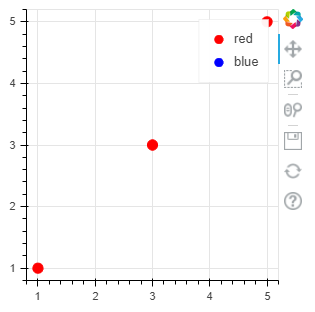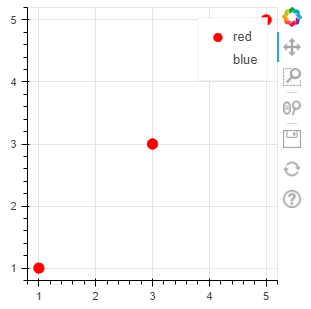# Legend glyph cannot be displayed if data is filtered out by CDSView

With Bokeh v2.2.3, the following codes can show the legend glyph if data is filtered out by CDSView.

``````source = ColumnDataSource(data=dict(x=[1, 2, 3, 4, 5], y=[1, 2, 3, 4, 5]))
view1 = CDSView(source=source, filters=[IndexFilter([0, 2, 4])])
view2 = CDSView(source=source, filters=[IndexFilter([])])

p = figure(height=300, width=300)
p.circle(x="x", y="y", size=10, color="red", legend_label="red", source=source, view=view1)
p.circle(x="x", y="y", size=10, color="blue", legend_label="blue", source=source, view=view2)

save(p)
``````However if we upgrade Bokeh to the latest version 2.4.1, the same codes produce the different result. The legend glyph is missing if the data is all filtered out by CDSView.(The blue circle is missing in the legend)

Is anything about legend settings changed between 2.2.3 and 2.4.1?

Seems like a bug, a GitHub Issue would be appropriate.

OK, thanks.
I’ll raise an issue on Github.